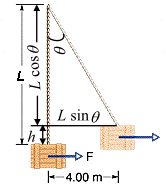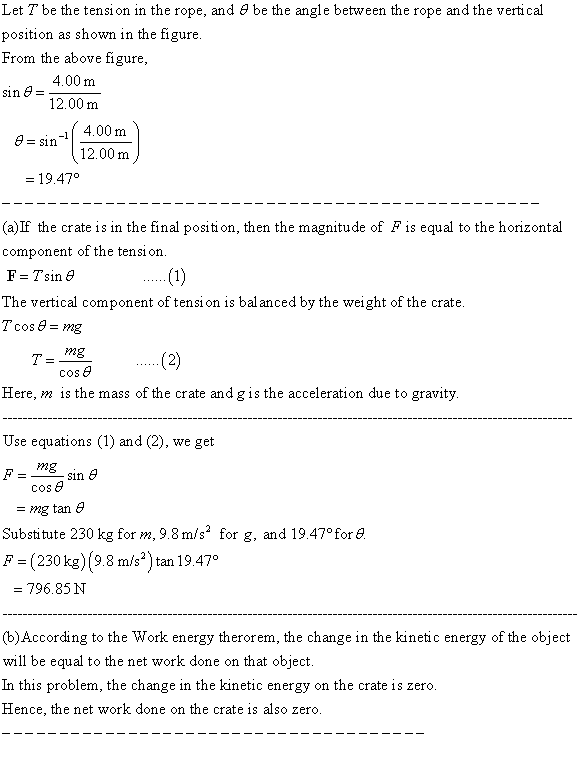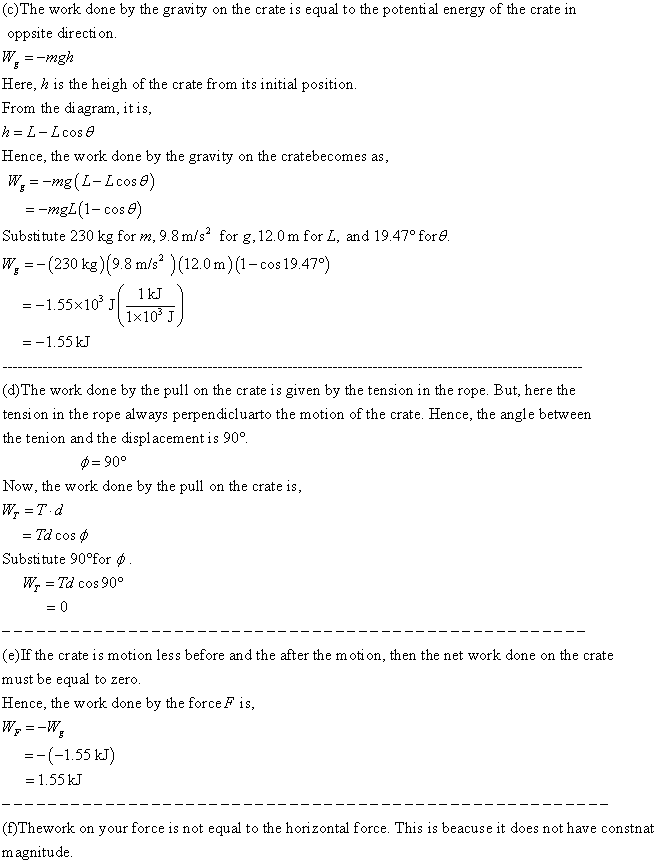# A 230kg crate hangs from the end of a rope of length L=12.0m. You push horizontally on the crate with a varying force F to move it distance d= 4.00m tallhvasstH 2021-02-10 Answered
A 230kg crate hangs from the end of a rope of length L=12.0m. You push horizontally on the crate with a varying force F to move it distance d= 4.00m to the side.
a) What is the magnitude of F when the crate is in this final position? What are b) the total work done on it, c) the work done by the gravitational force on the crate, and d) the work done by the pull on the crate fromthe rope? e) Knowing the the crate is motionless before and after its displacement, use the answers to (b), (c), and (d) to find the work your force F does on the crate. f) Why is the work of your force not equal to the product of the horizontal displacement and the answer to (a)?
You can still ask an expert for help

• Questions are typically answered in as fast as 30 minutes

Solve your problem for the price of one coffee

• Math expert for every subject
• Pay only if we can solve itDerrick

The free body diagram for the given system is shown in the following figure.